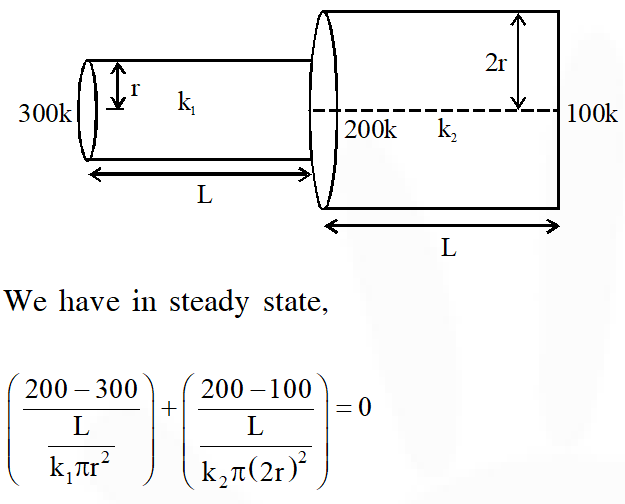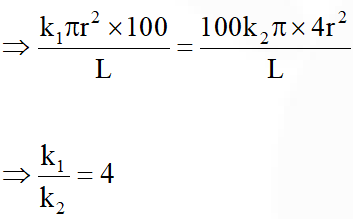Most Affordable JEE | NEET | 8,9,10 Preparation by Kota's Top IITian Doctor Faculties

# Heat Transfer - JEE Advanced Previous Year Questions with SolutionsJEE Advanced Previous Year Questions of Physics with Solutions are available at eSaral. Practicing JEE Advanced Previous Year Papers Questions of Physics will help the JEE aspirants in realizing the question pattern as well as help in analyzing weak & strong areas. Get detailed Class 11th & 12th Physics Notes to prepare for Boards as well as competitive exams like IIT JEE, NEET etc. eSaral helps the students in clearing and understanding each topic in a better way. eSaral is providing complete chapter-wise notes of Class 11th and 12th both for all subjects. Download eSaral app for free study material and video tutorials. Click Here for JEE main Previous Year Topic Wise Questions of Physics with Solutions  Simulator Previous Years JEE Advanced Questions
Q. A metal rod AB of length 10x has its one end A in ice at $0^{\circ}$C and the other end B in water at $100^{\circ}$C. If a point P on the rod is maintained at $400^{\circ}$C, then it is found that equal amounts of water and ice evaporate and melt per unit time. The latent heat of evaporation of water is 540 cal/g and latent heat of melting of ice is 80 cal/g. If the point P is at a distance of $\lambda \mathbf{x}$ from the ice end A, find the value of $\lambda$.[ Neglect any heat loss to the surrounding] [JEE 2009]
Ans.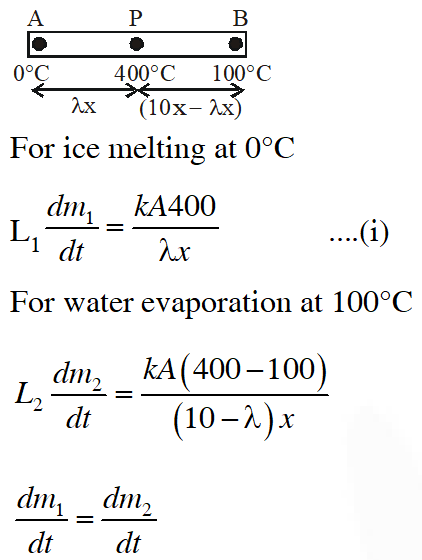Q. Two spherical bodies A (radius 6 cm) and B (radius 18 cm) are at temperatures $\mathrm{T}_{1}$ and $\mathrm{T}_{2}$, respectively. The maximum intensity in the emission spectrum of A is at 500 nm and in that of B is at 1500 nm. Considering then to be black bodies, what will be the ratio of the rate of total energy radiated by A to that of B ? [JEE 2010]
Ans. 9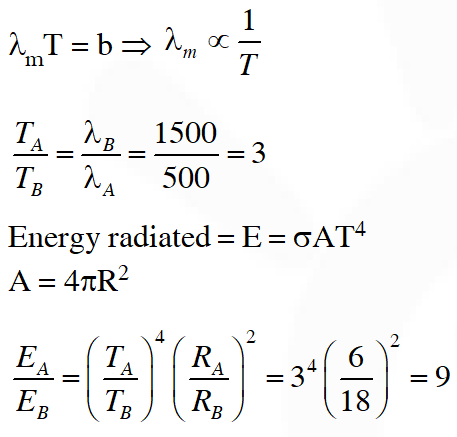Q. A composite block is made of slabs A, B, C, D and E of different thermal conductivities (given in terms of a constant K) and sizes (given in terms of length, L) as shown in the figure. All slabs are of same width. Heat ‘Q’ flows only from left to right through the blocks. Then in steady state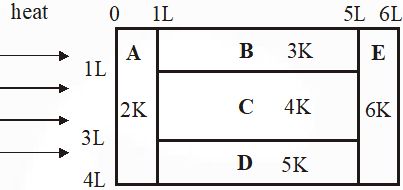(A) heat flow through A and E slabs are same (B) heat flow through slab E is maximum (C) temperature difference across slab E is smallest (D) heat flow through C= heat flow through B + Heat flow through D [JEE 2011]
Ans. (A, C, D or A, B,C,D)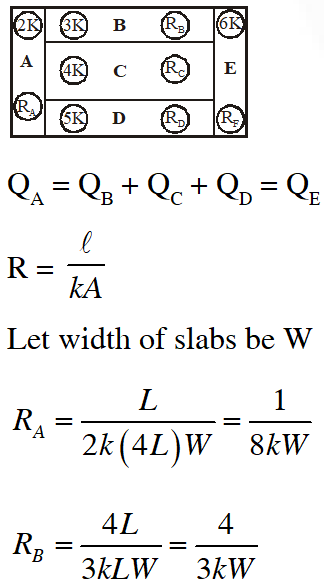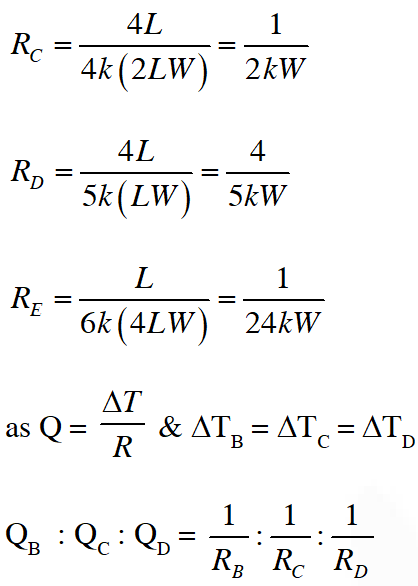Q. Three very large plates of same area are kept parallel and close to each other. They are considered as ideal black surfaces and have very high thermal conductivity. The first and third plates are maintained at temperatures 2T and 3T respectively. The temperature of the middle (i.e. second) plate under steady state condition is $(\mathrm{A})\left(\frac{65}{2}\right)^{1 / 4} T$ $(\mathrm{B})\left(\frac{97}{4}\right)^{1 / 4} T$ $(\mathrm{C})\left(\frac{97}{2}\right)^{1 / 4} T$ (D) $(97)^{1 / 4} T$ [JEE 2012]
Ans. (C)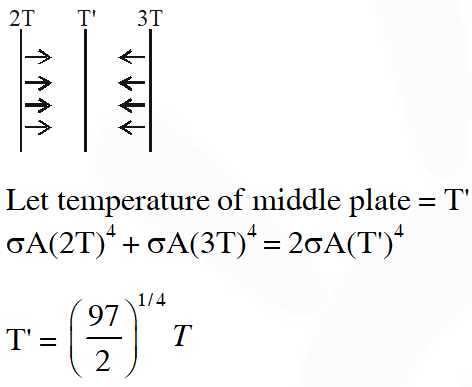Q. Two rectangular blocks, having identical dimensions, can be arranged either in configuration I or in configuration II as shown in the figure. One of the blocks has thermal conductivity k and the other 2k. The temperature difference between the ends along the x-axis is the same in both the configurations. It takes 9s to transport a certain amount of heat from the hot end to the cold end in the configuration I. The time to transport the same amount of heat in the configuration II is :-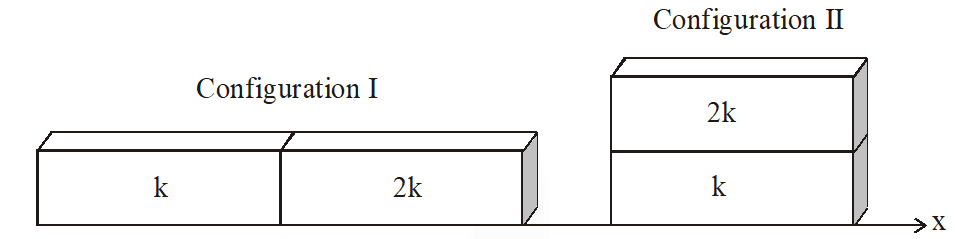(A) 2.0 s           (B) 3.0 s            (C) 4.5 s            (D) 6.0 s [JEE-Advance-2013]
Ans. (A)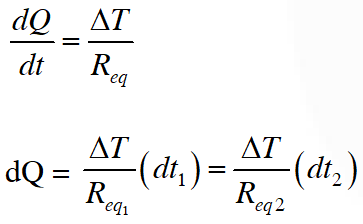Q. Parallel rays of light of intensity I = 912 $\mathrm{W} \mathrm{m}^{-2}$ are incident on a spherical black body kept in surroundings of temperature 300 K. Take Stefan-Boltzmann constant $\sigma=5.7 \times 10^{-8} \mathrm{Wm}^{-2} \mathrm{K}^{-4}$and assume that the energy exchange with the surroundings is only through radiation. The final steady state temperature of the black body is close to :- (A) 330 K (B) 660 K (C) 990 K (D) 1550 K [JEE-Advance-2014]
Ans. (A)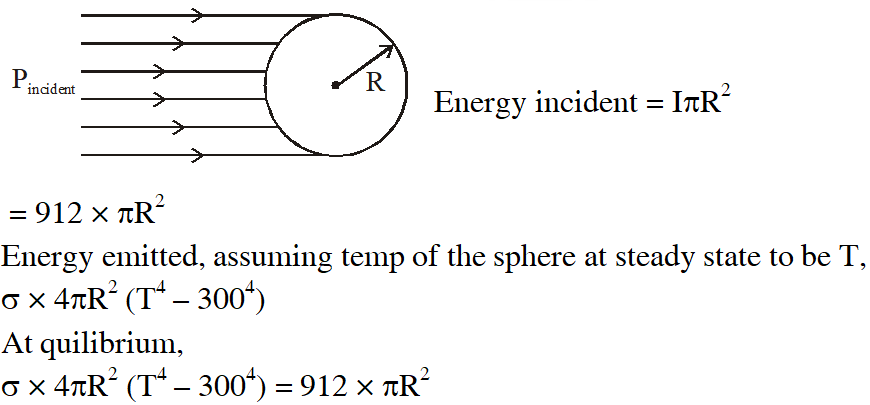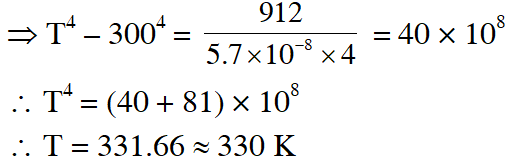Q. Two spherical stars A and B emit blackbody radiation. The radius of A is 400 times that of B and A emits $10^{4}$ times the power emitted from B. The ratio $\left(\frac{\lambda_{\mathrm{A}}}{\lambda_{\mathrm{B}}}\right)$ of their wavelengths $\lambda_{\mathrm{A}}$ and $\lambda_{\mathrm{B}}$at which the peaks occur in their respective radiation curves is. [JEE-Advance-2015]
Ans. 2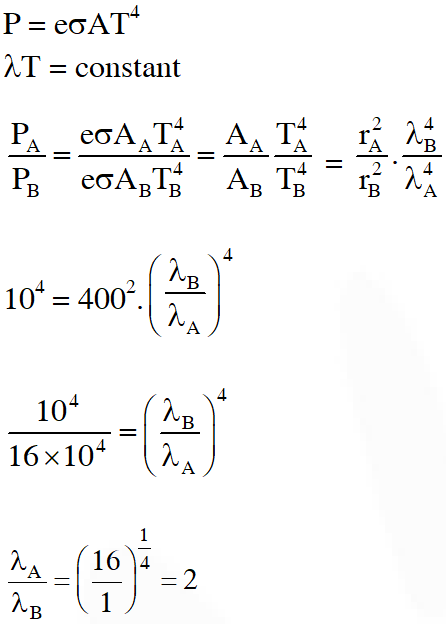Q. A metal is heated in a furnace where a sensor is kept above the metal surface to read the power radiated (P) by the metal. The sensor has a scale that displays $\log _{2}\left(\mathrm{P} / \mathrm{P}_{0}\right)$, where $P_{0}$ is a constant. When the metal surface is at a temperature of $487^{\circ} \mathrm{C}$, the sensor shows a value 1. Assume that the emissivity of the metallic surface remains constant. What is the value displayed by the sensor when the temperature of the metal surface is raised to $2767^{\circ} \mathrm{C}$ ? [JEE-Advance-2016]
Ans. 9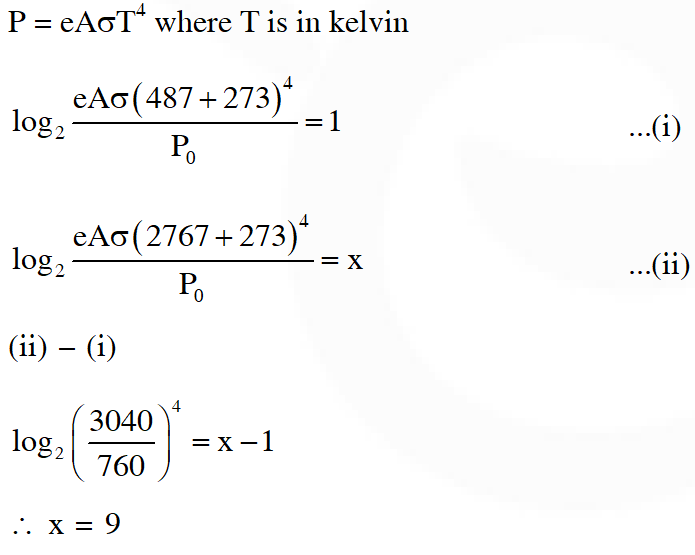Q. The ends Q and R of two thin wires, PQ and RS, are soldered (joined) together. Initially each of the wires has a length of 1m at $10^{\circ} \mathrm{C}$. Now the end P is maintained at $10^{\circ}$C, while the end S is heated and maintained at $400^{\circ} \mathrm{C}$. The system is thermally insulated from its surroundings. If the thermal conductivity of wire PQ is twice that of the wire RS and the coefficient of linear thermal expansion of PQ is $1.2 \times 10^{-5} \mathrm{K}^{-1}$, the change in length of the wire PQ is : - (A) 0.78 mm (B) 0.90 mm (C) 1.56 mm (D) 2.34 mm [JEE-Advance-2016]
Ans. (A)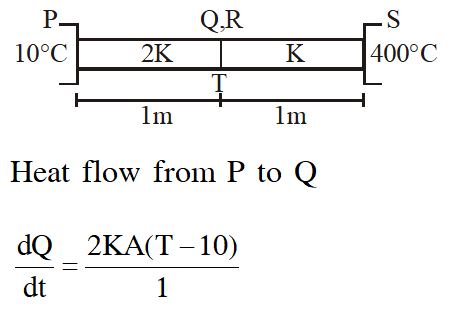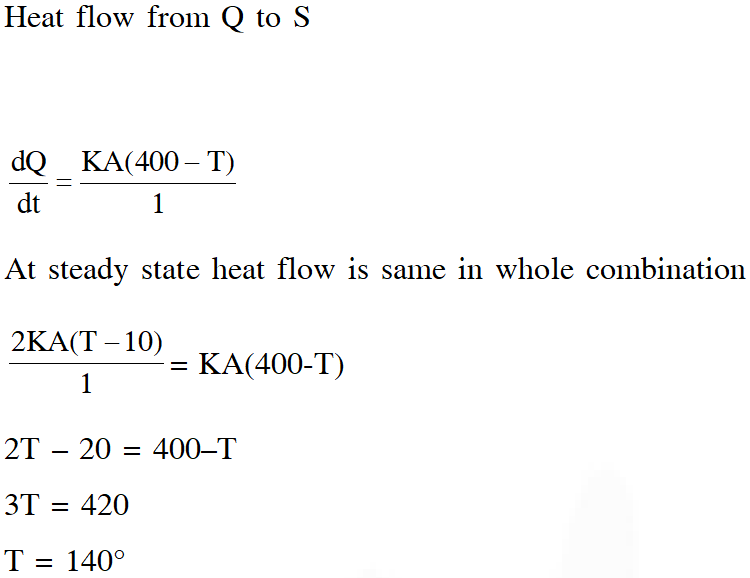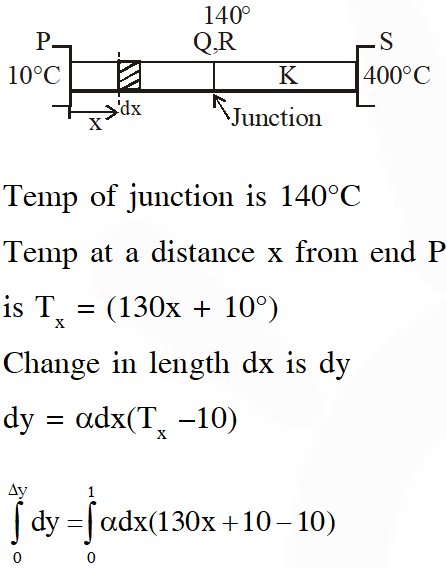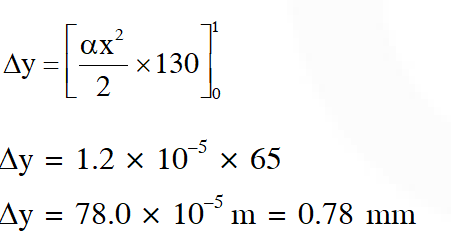Q. A human body has a surface area of approximately 1 $\mathrm{m}^{2}$. The normal body temperature is 10 K above the surrounding room temperature T0. Take the room temperature to be $\mathrm{T}_{0}=300 \mathrm{K} .$ For $\mathrm{T}_{0}=300 \mathrm{K}$, the value of = $460 \mathrm{Wm}^{-2}$ (where $\sigma$ is the Stefan-Boltzmann constant). Which of the following options is/are correct ? (A) The amount of energy radiated by the body in 1 second is close to 60 Joules (B) If the surrounding temperature reduces by a small amount $\Delta \mathrm{T}_{0}<<\mathrm{T}_{0}$, then to maintain the same body temperature the same (living) human being needs to radiate $\Delta \mathrm{W}$ = more energy per unit time (C) Reducing the exposed surface area of the body (e.g. by curling up) allows humans to maintain the same body temperature while reducing the energy lost by radiation (D) If the body temperature rises significantly then the peak in the spectrum of electromagnetic radiation emitted by the body would shift to longer wavelengths [JEE-Advance-2017]
Ans. (A,B,C) or (C)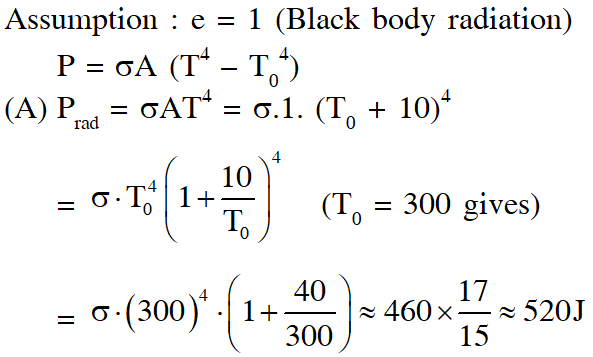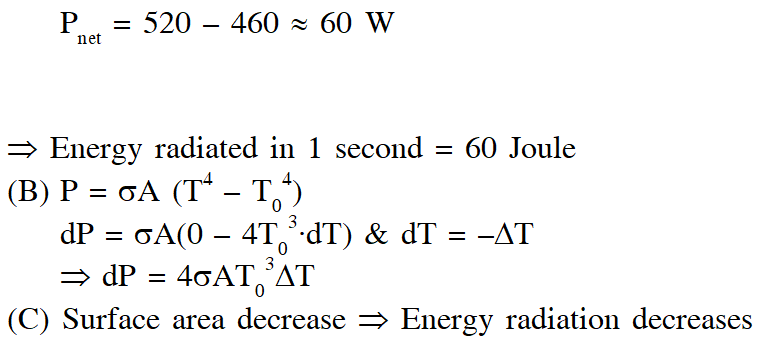Q. Two conducting cylinders of equal length but different radii are connected in series between two heat baths kept at temperatures $\mathrm{T}_{1}=300 \mathrm{K}$ and $\mathrm{T}_{2}=100 \mathrm{K}$K, as shown in the figure. The radius of the bigger cylinder is twice that of the smaller one and the thermal conductivities of the materials of the smaller and the larger cylinders are $\mathrm{K}_{1}$ and $\mathrm{K}_{2}$ respectively. If the temperature at the junction of the two cylinders in the steady state is 200 K, then $\mathrm{K}_{1} / \mathrm{K}_{2}$ = _________.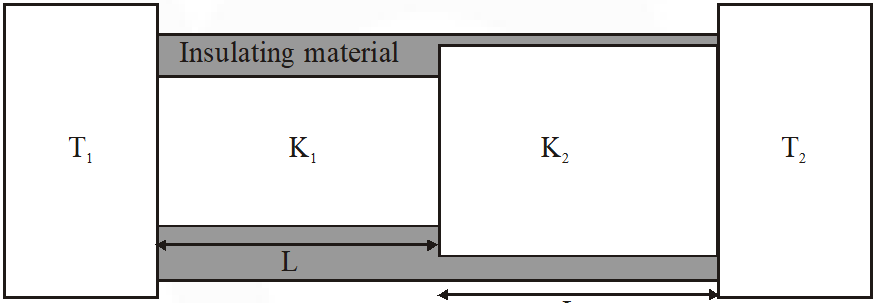[JEE-Advance-2018]
Ans. 4